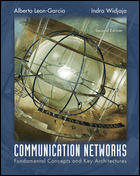{itemname} {itemname}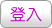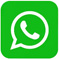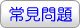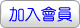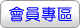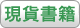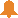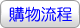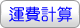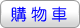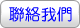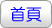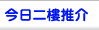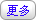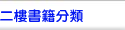PROBABILITY, STATISTICS, AND RANDOM PROCESSES FOR ELECTRICAL ENGINEERING 3/E (PIE)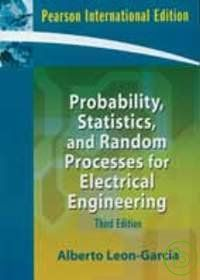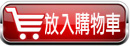沒有庫存 訂購需時10-14天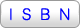9780132336215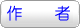LEON-GARCIA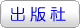全華科技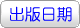2008年1月01日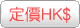417.00  元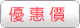HK\$ 396.15省下 \$20.85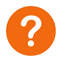* 叢書系列：大專電子
* 規格：平裝 / 832頁 / 普級 / 單色印刷 / 初版
* 出版地：台灣

[ 尚未分類 ]

While helping students to develop their problem-solving skills, the author motivates students with practical applications from various areas of ECE that demonstrate the relevance of probability theory to engineering practice.

1 . Chapter overviews: brief introduction outlining chapter coverage and learning objectives.
2 . Chapter summaries: concise, easy-reference sections providing quick overviews of each chapter’’s major topics.
3 . Checklist of important terms.
4 . Annotated references: suggestions of timely resources for additional coverage of critical material.
5 . Numerous examples: a wide selection of fully worked-out real-world examples.
6 . Computer Methods sections have been updated and substantially enhanced
7 . New problems have been added

1. Probability Models in Electrical and Computer Engineering
2. Basic Concepts of Probability Theory
3. Discrete Random Variables
4. One Random Variable
5. Pairs of Random Variables
6. Vector Random Variables
7. Sums of Random Variables and Long-Term Averages
8. Statistics
9. Random Processes
10. Analysis and Processing of Random Signals
11. Markov Chains
12. Introduction to Queueing Theory
Appendix A. Mathematical Tables
Appendix B. Tables of Fourier Transformation
Appendix C. Computer Programs for Generating Random Variables
Index

 其 他 著 作 1. Communication Networks